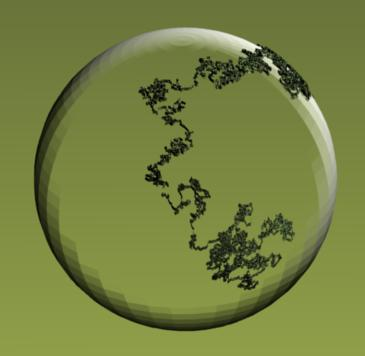# 另一个视角深度看随机过程Stochastic Process

## 随机变量与其分布

“我们可以用一个概率A去刻画投硬币后落下正面朝上的可能性，用另一个概率B去刻画投硬币落下后反面朝上的可能性”。

*我们可以用随机变量其所有可能发生的情况与其所对应的可能性的联合去刻画一个随机变量！*

$P(投币结果=正面) = A$, $P(投币结果=反面)=B$

Okay，现在我们稍稍总结一下刚才的例子，并且抽象一下。

Bingo！

$P(投币1结果=正面, 投币2的结果=正面) = A$,

$P(投币1结果=反面, 投币2的结果=正面) = B$,

$P(投币1结果=正面, 投币2的结果=反面) = C$,

$P(投币1结果=反面, 投币2的结果=反面) = D$,

## 随机过程与随机向量有什么不同？##### 蓦风星吟
###### 文艺小资的数学PhD/助理教授

Mathematics + Data + Me = Magic